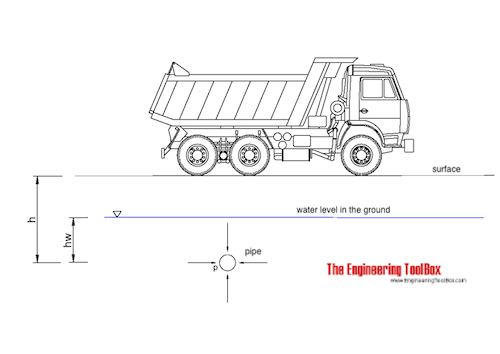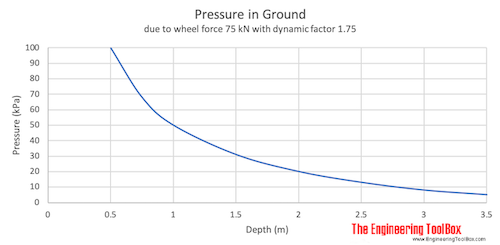Engineering ToolBox - Resources, Tools and Basic Information for Engineering and Design of Technical Applications!

# Underground Pipes - Earth Pressure

## Pressure acting on underground pipes due to weight of soil and transport.The pressure acting on an object in the ground - typical on the outside of a pipe can be calculated as

p = psoil + pwater + ptransport               (1)

where

p = pressure acting on object in the ground (Pa)

psoil = pressure caused by the weight of the soil above the object (Pa)

pwater = hydrostatic pressure caused by the weight of the water above the object (Pa)

ptransport = pressure caused by the transport load on surface of the soil (Pa)

• 1 Pa = 10-6 N/mm2 = 10-3 kPa = 1.450x10-4 psi (lbf/in2)

Pressure caused by soil

psoil = ρs g (h - hw) + ρsw g hw                 (2)

where

ρs = density of soil above the groundwater (typical 1800 - 1900 kg/m3)

g = acceleration of gravity (9.81 m/s2)

h = depth from surface to object (m)

hw = depth from groundwater level to object (m)

ρswdensity of soil below groundwater level (reduced due to Buoyancy of soil - typical value 1100 kg/m3)

Pressure caused by groundwater

pwater = ρw g hw                 (3)

where

ρsw = density water  (1000 kg/m3)

Pressure caused by transport

The pressure caused by transport depends on the force (weight) on each wheel. The figure below indicates the the pressure at different depths for wheel force 75 kN (7500 kg) with dynamic factor 1.75.### Example - Pressure acting on an Underground Pipe

The pressure acting on pipe at buried at depth 2 m and 2 m below groundwater level can be calculated as

psoil = [(1800 kg/m3) (9.81 m/s2) ((2 m) - (1 m)) ] + [(1100 kg/m3(9.81 m/s2) (1 m)]

= 28449 Pa

= 28.5 kPa

pwater = (1000 kg/m3(9.81 m/s2) (1 m)

= 9810 Pa

= 9.8 kPa

ptransport = aprox. from the diagram  - 20 kPa

Total pressure

p = (28.5 kPa) + (9.8 kPa) + (20 kPa)

= 58 kPa

• 1 kPa = 1 kN/m2

### Underground Pressure Calculator

This calculator is based on the equations below and can used to estimate the underground pressure acting on objects relatively smaller than the depth they are buried - typically pipes with diameter less than 3-500 mm.

Pressure from transport is calculated for wheel force 75 kN (7500 kg) with dynamic factor 1.75.

density of soil (kg/m3)

density of soil below groundwater (kg/m3)

- depth from surface to object (m)

- depth from groundwater level to object (m)

## Related Topics

• Miscellaneous - Engineering related topics like Beaufort Wind Scale, CE-marking, drawing standards and more.

## Engineering ToolBox - SketchUp Extension - Online 3D modeling!

Add standard and customized parametric components - like flange beams, lumbers, piping, stairs and more - to your Sketchup model with the Engineering ToolBox - SketchUp Extension - enabled for use with the amazing, fun and free SketchUp Make and SketchUp Pro .Add the Engineering ToolBox extension to your SketchUp from the SketchUp Pro Sketchup Extension Warehouse!

Translate

## Privacy

We don't collect information from our users. Only emails and answers are saved in our archive. Cookies are only used in the browser to improve user experience.

Some of our calculators and applications let you save application data to your local computer. These applications will - due to browser restrictions - send data between your browser and our server. We don't save this data.

## Citation

• Engineering ToolBox, (2018). Underground Pipes - Earth Pressure. [online] Available at: https://www.engineeringtoolbox.com/underground-pipe-pressure-soil-transport-d_2145.html [Accessed Day Mo. Year].

Modify access date.

. .

#### Scientific Online Calculator6 24